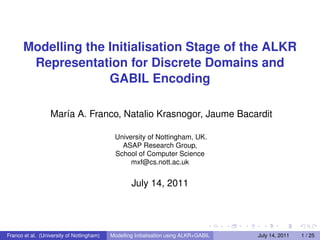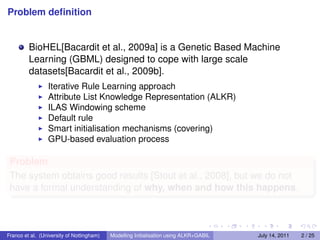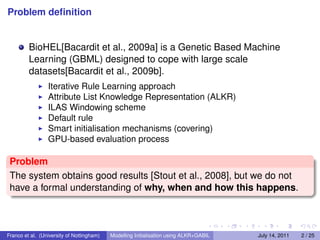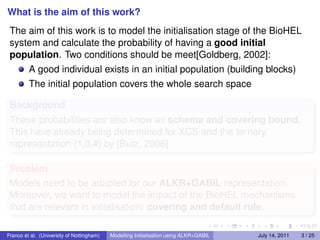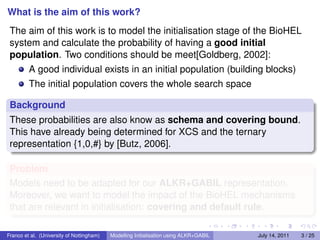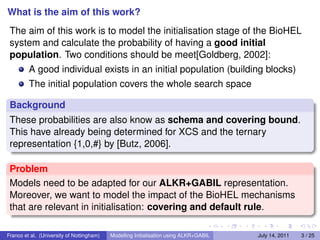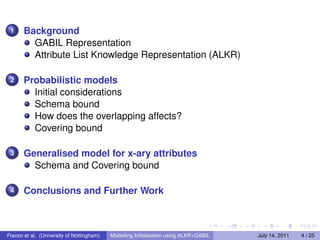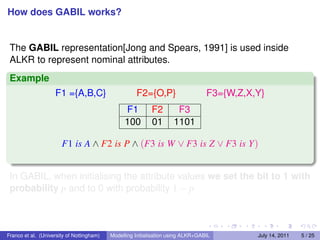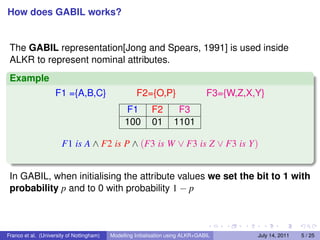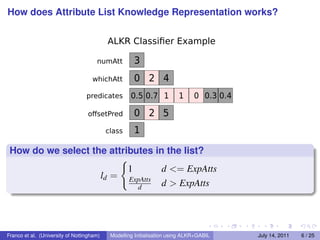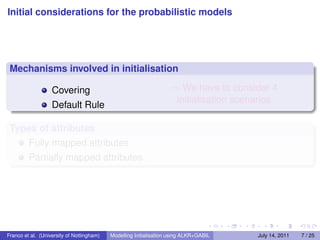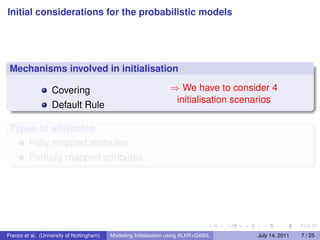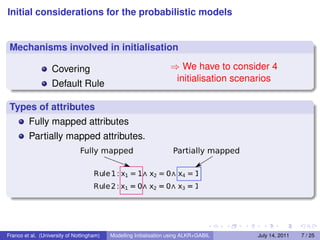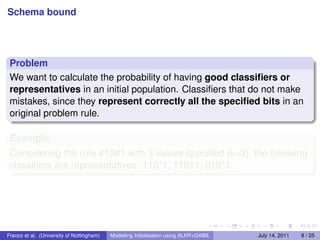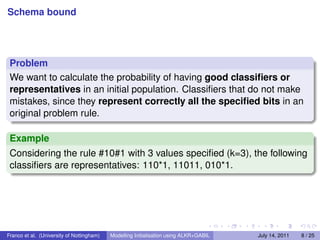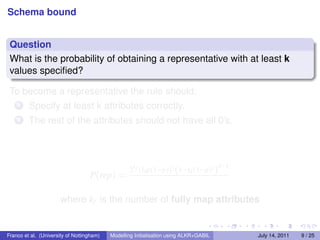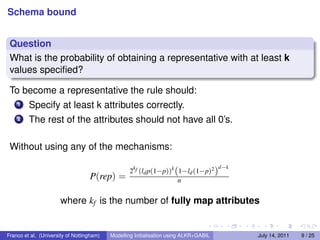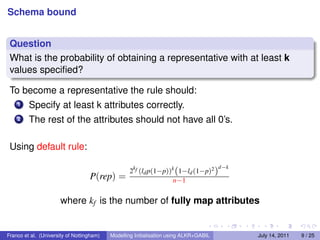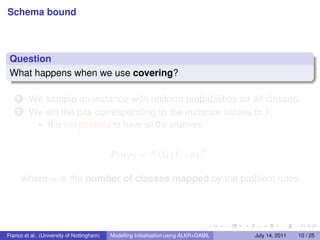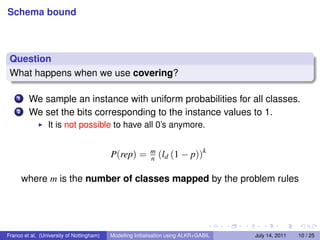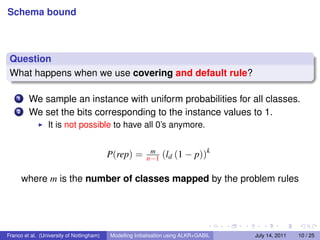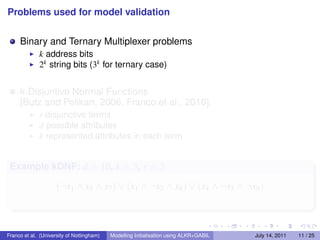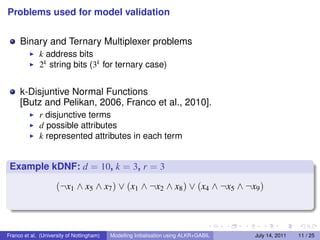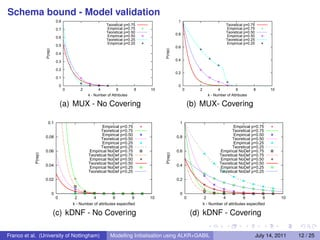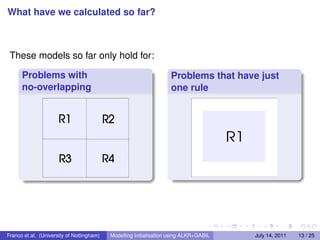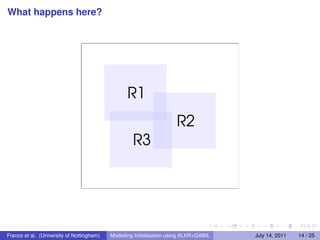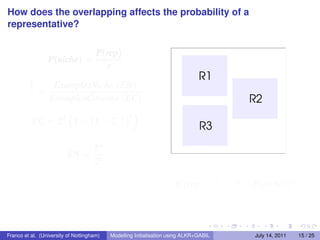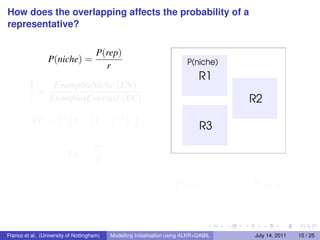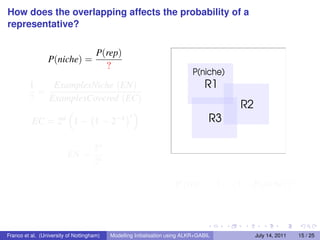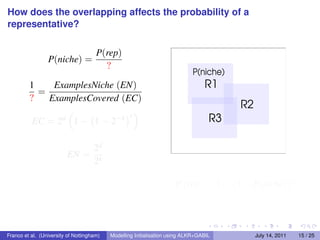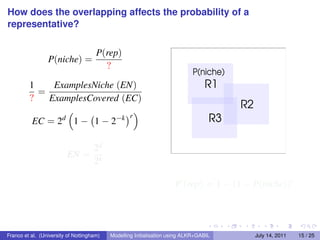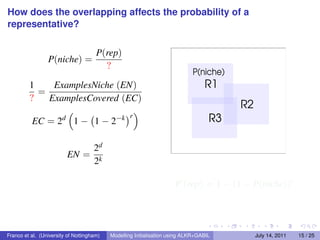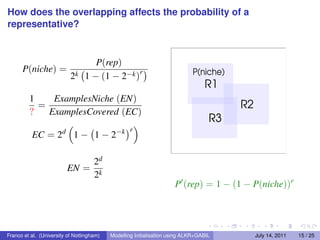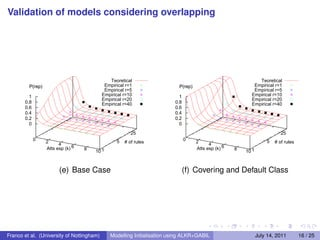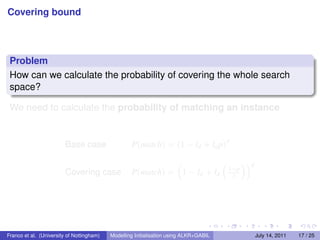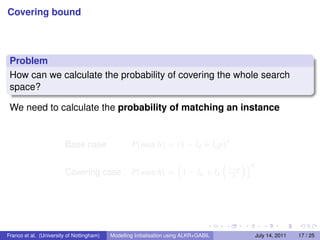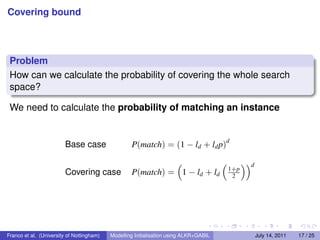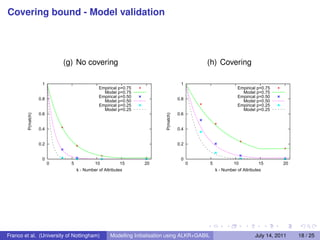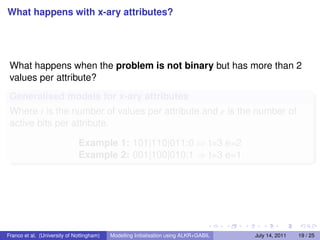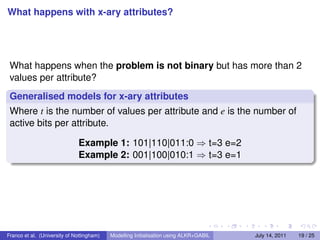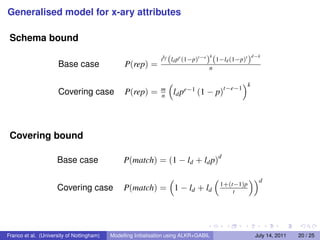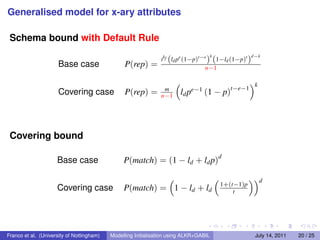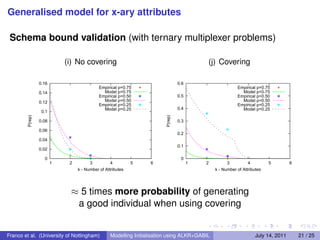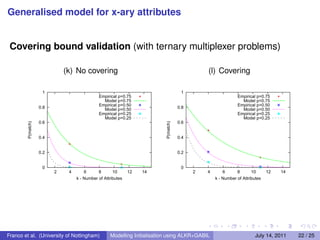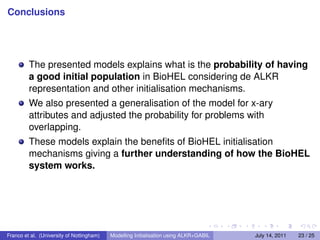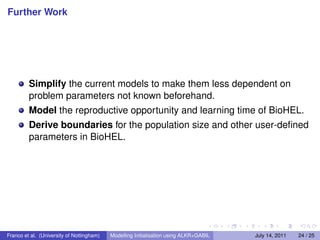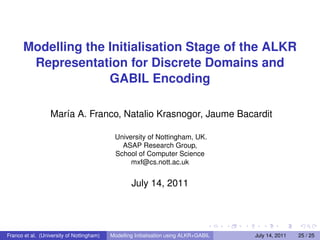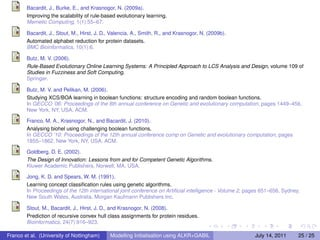1 of 48

### Modelling the Initialisation Stage of the ALKR Representation for Discrete Domains and GABIL Encoding

1. Modelling the Initialisation Stage of the ALKR Representation for Discrete Domains and GABIL Encoding María A. Franco, Natalio Krasnogor, Jaume Bacardit University of Nottingham, UK. ASAP Research Group, School of Computer Science mxf@cs.nott.ac.uk July 14, 2011 Franco et al. (University of Nottingham) Modelling Initialisation using ALKR+GABIL July 14, 2011 1 / 25
2. Problem deﬁnition BioHEL[Bacardit et al., 2009a] is a Genetic Based Machine Learning (GBML) designed to cope with large scale datasets[Bacardit et al., 2009b]. Iterative Rule Learning approach Attribute List Knowledge Representation (ALKR) ILAS Windowing scheme Default rule Smart initialisation mechanisms (covering) GPU-based evaluation process Problem The system obtains good results [Stout et al., 2008], but we do not have a formal understanding of why, when and how this happens. Franco et al. (University of Nottingham) Modelling Initialisation using ALKR+GABIL July 14, 2011 2 / 25
3. Problem deﬁnition BioHEL[Bacardit et al., 2009a] is a Genetic Based Machine Learning (GBML) designed to cope with large scale datasets[Bacardit et al., 2009b]. Iterative Rule Learning approach Attribute List Knowledge Representation (ALKR) ILAS Windowing scheme Default rule Smart initialisation mechanisms (covering) GPU-based evaluation process Problem The system obtains good results [Stout et al., 2008], but we do not have a formal understanding of why, when and how this happens. Franco et al. (University of Nottingham) Modelling Initialisation using ALKR+GABIL July 14, 2011 2 / 25
4. What is the aim of this work? The aim of this work is to model the initialisation stage of the BioHEL system and calculate the probability of having a good initial population. Two conditions should be meet[Goldberg, 2002]: A good individual exists in an initial population (building blocks) The initial population covers the whole search space Background These probabilities are also know as schema and covering bound. This have already being determined for XCS and the ternary representation {1,0,#} by [Butz, 2006]. Problem Models need to be adapted for our ALKR+GABIL representation. Moreover, we want to model the impact of the BioHEL mechanisms that are relevant in initialisation: covering and default rule. Franco et al. (University of Nottingham) Modelling Initialisation using ALKR+GABIL July 14, 2011 3 / 25
5. What is the aim of this work? The aim of this work is to model the initialisation stage of the BioHEL system and calculate the probability of having a good initial population. Two conditions should be meet[Goldberg, 2002]: A good individual exists in an initial population (building blocks) The initial population covers the whole search space Background These probabilities are also know as schema and covering bound. This have already being determined for XCS and the ternary representation {1,0,#} by [Butz, 2006]. Problem Models need to be adapted for our ALKR+GABIL representation. Moreover, we want to model the impact of the BioHEL mechanisms that are relevant in initialisation: covering and default rule. Franco et al. (University of Nottingham) Modelling Initialisation using ALKR+GABIL July 14, 2011 3 / 25
6. What is the aim of this work? The aim of this work is to model the initialisation stage of the BioHEL system and calculate the probability of having a good initial population. Two conditions should be meet[Goldberg, 2002]: A good individual exists in an initial population (building blocks) The initial population covers the whole search space Background These probabilities are also know as schema and covering bound. This have already being determined for XCS and the ternary representation {1,0,#} by [Butz, 2006]. Problem Models need to be adapted for our ALKR+GABIL representation. Moreover, we want to model the impact of the BioHEL mechanisms that are relevant in initialisation: covering and default rule. Franco et al. (University of Nottingham) Modelling Initialisation using ALKR+GABIL July 14, 2011 3 / 25
7. 1 Background GABIL Representation Attribute List Knowledge Representation (ALKR) 2 Probabilistic models Initial considerations Schema bound How does the overlapping affects? Covering bound 3 Generalised model for x-ary attributes Schema and Covering bound 4 Conclusions and Further Work Franco et al. (University of Nottingham) Modelling Initialisation using ALKR+GABIL July 14, 2011 4 / 25
8. How does GABIL works? The GABIL representation[Jong and Spears, 1991] is used inside ALKR to represent nominal attributes. Example F1 ={A,B,C} F2={O,P} F3={W,Z,X,Y} F1 F2 F3 100 01 1101 F1 is A ∧ F2 is P ∧ (F3 is W ∨ F3 is Z ∨ F3 is Y) In GABIL, when initialising the attribute values we set the bit to 1 with probability p and to 0 with probability 1 − p Franco et al. (University of Nottingham) Modelling Initialisation using ALKR+GABIL July 14, 2011 5 / 25
9. How does GABIL works? The GABIL representation[Jong and Spears, 1991] is used inside ALKR to represent nominal attributes. Example F1 ={A,B,C} F2={O,P} F3={W,Z,X,Y} F1 F2 F3 100 01 1101 F1 is A ∧ F2 is P ∧ (F3 is W ∨ F3 is Z ∨ F3 is Y) In GABIL, when initialising the attribute values we set the bit to 1 with probability p and to 0 with probability 1 − p Franco et al. (University of Nottingham) Modelling Initialisation using ALKR+GABIL July 14, 2011 5 / 25
10. How does Attribute List Knowledge Representation works? ALKR Classiﬁer Example numAtt 3 whichAtt 0 predicates 0.5 0.7 0.3 oﬀsetPred 0 class 1 How do we select the attributes in the list? 1 d <= ExpAtts ld = ExpAtts d d > ExpAtts Franco et al. (University of Nottingham) Modelling Initialisation using ALKR+GABIL July 14, 2011 6 / 25
11. Initial considerations for the probabilistic models Mechanisms involved in initialisation Covering ⇒ We have to consider 4 initialisation scenarios Default Rule Types of attributes Fully mapped attributes Partially mapped attributes. Franco et al. (University of Nottingham) Modelling Initialisation using ALKR+GABIL July 14, 2011 7 / 25
12. Initial considerations for the probabilistic models Mechanisms involved in initialisation Covering ⇒ We have to consider 4 initialisation scenarios Default Rule Types of attributes Fully mapped attributes Partially mapped attributes. Franco et al. (University of Nottingham) Modelling Initialisation using ALKR+GABIL July 14, 2011 7 / 25
13. Initial considerations for the probabilistic models Mechanisms involved in initialisation Covering ⇒ We have to consider 4 initialisation scenarios Default Rule Types of attributes Fully mapped attributes Partially mapped attributes. Franco et al. (University of Nottingham) Modelling Initialisation using ALKR+GABIL July 14, 2011 7 / 25
14. Schema bound Problem We want to calculate the probability of having good classiﬁers or representatives in an initial population. Classiﬁers that do not make mistakes, since they represent correctly all the speciﬁed bits in an original problem rule. Example Considering the rule #10#1 with 3 values speciﬁed (k=3), the following classiﬁers are representatives: 110*1, 11011, 010*1. Franco et al. (University of Nottingham) Modelling Initialisation using ALKR+GABIL July 14, 2011 8 / 25
15. Schema bound Problem We want to calculate the probability of having good classiﬁers or representatives in an initial population. Classiﬁers that do not make mistakes, since they represent correctly all the speciﬁed bits in an original problem rule. Example Considering the rule #10#1 with 3 values speciﬁed (k=3), the following classiﬁers are representatives: 110*1, 11011, 010*1. Franco et al. (University of Nottingham) Modelling Initialisation using ALKR+GABIL July 14, 2011 8 / 25
16. Schema bound Question What is the probability of obtaining a representative with at least k values speciﬁed? To become a representative the rule should: 1 Specify at least k attributes correctly. 2 The rest of the attributes should not have all 0’s. k d−k 2 f (ld p(1−p))k (1−ld (1−p)2 ) P(rep) = where kf is the number of fully map attributes Franco et al. (University of Nottingham) Modelling Initialisation using ALKR+GABIL July 14, 2011 9 / 25
17. Schema bound Question What is the probability of obtaining a representative with at least k values speciﬁed? To become a representative the rule should: 1 Specify at least k attributes correctly. 2 The rest of the attributes should not have all 0’s. Without using any of the mechanisms: k d−k 2 f (ld p(1−p))k (1−ld (1−p)2 ) P(rep) = n where kf is the number of fully map attributes Franco et al. (University of Nottingham) Modelling Initialisation using ALKR+GABIL July 14, 2011 9 / 25
18. Schema bound Question What is the probability of obtaining a representative with at least k values speciﬁed? To become a representative the rule should: 1 Specify at least k attributes correctly. 2 The rest of the attributes should not have all 0’s. Using default rule: k d−k 2 f (ld p(1−p))k (1−ld (1−p)2 ) P(rep) = n−1 where kf is the number of fully map attributes Franco et al. (University of Nottingham) Modelling Initialisation using ALKR+GABIL July 14, 2011 9 / 25
19. Schema bound Question What happens when we use covering? 1 We sample an instance with uniform probabilities for all classes. 2 We set the bits corresponding to the instance values to 1. It is not possible to have all 0’s anymore. P(rep) = m (ld (1 − p))k where m is the number of classes mapped by the problem rules Franco et al. (University of Nottingham) Modelling Initialisation using ALKR+GABIL July 14, 2011 10 / 25
20. Schema bound Question What happens when we use covering? 1 We sample an instance with uniform probabilities for all classes. 2 We set the bits corresponding to the instance values to 1. It is not possible to have all 0’s anymore. P(rep) = m n (ld (1 − p))k where m is the number of classes mapped by the problem rules Franco et al. (University of Nottingham) Modelling Initialisation using ALKR+GABIL July 14, 2011 10 / 25
21. Schema bound Question What happens when we use covering and default rule? 1 We sample an instance with uniform probabilities for all classes. 2 We set the bits corresponding to the instance values to 1. It is not possible to have all 0’s anymore. P(rep) = m n−1 (ld (1 − p))k where m is the number of classes mapped by the problem rules Franco et al. (University of Nottingham) Modelling Initialisation using ALKR+GABIL July 14, 2011 10 / 25
22. Problems used for model validation Binary and Ternary Multiplexer problems k address bits 2k string bits (3k for ternary case) k-Disjuntive Normal Functions [Butz and Pelikan, 2006, Franco et al., 2010]. r disjunctive terms d possible attributes k represented attributes in each term Example kDNF: d = 10, k = 3, r = 3 (¬x1 ∧ x5 ∧ x7 ) ∨ (x1 ∧ ¬x2 ∧ x8 ) ∨ (x4 ∧ ¬x5 ∧ ¬x9 ) Franco et al. (University of Nottingham) Modelling Initialisation using ALKR+GABIL July 14, 2011 11 / 25
23. Problems used for model validation Binary and Ternary Multiplexer problems k address bits 2k string bits (3k for ternary case) k-Disjuntive Normal Functions [Butz and Pelikan, 2006, Franco et al., 2010]. r disjunctive terms d possible attributes k represented attributes in each term Example kDNF: d = 10, k = 3, r = 3 (¬x1 ∧ x5 ∧ x7 ) ∨ (x1 ∧ ¬x2 ∧ x8 ) ∨ (x4 ∧ ¬x5 ∧ ¬x9 ) Franco et al. (University of Nottingham) Modelling Initialisation using ALKR+GABIL July 14, 2011 11 / 25
24. Schema bound - Model validation 0.8 1 Teoretical p=0.75 Teoretical p=0.75 0.7 Empirical p=0.75 Empirical p=0.75 Teoretical p=0.50 Teoretical p=0.50 Empirical p=0.50 0.8 Empirical p=0.50 0.6 Teoretical p=0.25 Teoretical p=0.25 Empirical p=0.25 Empirical p=0.25 0.5 0.6 P(rep) P(rep) 0.4 0.3 0.4 0.2 0.2 0.1 0 0 0 2 4 6 8 10 0 2 4 6 8 10 k - Number of Attributes k - Number of Attributes (a) MUX - No Covering (b) MUX- Covering 0.1 1 Empirical p=0.75 Empirical p=0.75 Teoretical p=0.75 Teoretical p=0.75 Empirical p=0.50 Empirical p=0.50 0.08 Teoretical p=0.50 0.8 Teoretical p=0.50 Empirical p=0.25 Empirical p=0.25 Teoretical p=0.25 Teoretical p=0.25 0.06 Empirical NoDef p=0.75 0.6 Empirical NoDef p=0.75 P(rep) P(rep) Teoretical NoDef p=0.75 Teoretical NoDef p=0.75 Empirical NoDef p=0.50 Empirical NoDef p=0.50 0.04 Teoretical NoDef p=0.50 0.4 Teoretical NoDef p=0.50 Empirical NoDef p=0.25 Empirical NoDef p=0.25 Teoretical NoDef p=0.25 Teoretical NoDef p=0.25 0.02 0.2 0 0 0 2 4 6 8 10 0 2 4 6 8 10 k - Number of attributes especified k - Number of attributes especified (c) kDNF - No Covering (d) kDNF - Covering Franco et al. (University of Nottingham) Modelling Initialisation using ALKR+GABIL July 14, 2011 12 / 25
25. What have we calculated so far? These models so far only hold for: Problems with Problems that have just no-overlapping one rule Franco et al. (University of Nottingham) Modelling Initialisation using ALKR+GABIL July 14, 2011 13 / 25
26. What happens here? Franco et al. (University of Nottingham) Modelling Initialisation using ALKR+GABIL July 14, 2011 14 / 25
27. How does the overlapping affects the probability of a representative? P(rep) P(niche) = r 1 ExamplesNiche (EN) = ? ExamplesCovered (EC) r EC = 2d 1 − 1 − 2−k 2d EN = 2k P (rep) = 1 − (1 − P(niche))r Franco et al. (University of Nottingham) Modelling Initialisation using ALKR+GABIL July 14, 2011 15 / 25
28. How does the overlapping affects the probability of a representative? P(rep) P(niche) = r 1 ExamplesNiche (EN) = ? ExamplesCovered (EC) r EC = 2d 1 − 1 − 2−k 2d EN = 2k P (rep) = 1 − (1 − P(niche))r Franco et al. (University of Nottingham) Modelling Initialisation using ALKR+GABIL July 14, 2011 15 / 25
29. How does the overlapping affects the probability of a representative? P(rep) P(niche) = ? 1 ExamplesNiche (EN) = ? ExamplesCovered (EC) r EC = 2d 1 − 1 − 2−k 2d EN = 2k P (rep) = 1 − (1 − P(niche))r Franco et al. (University of Nottingham) Modelling Initialisation using ALKR+GABIL July 14, 2011 15 / 25
30. How does the overlapping affects the probability of a representative? P(rep) P(niche) = ? 1 ExamplesNiche (EN) = ? ExamplesCovered (EC) r EC = 2d 1 − 1 − 2−k 2d EN = 2k P (rep) = 1 − (1 − P(niche))r Franco et al. (University of Nottingham) Modelling Initialisation using ALKR+GABIL July 14, 2011 15 / 25
31. How does the overlapping affects the probability of a representative? P(rep) P(niche) = ? 1 ExamplesNiche (EN) = ? ExamplesCovered (EC) r EC = 2d 1 − 1 − 2−k 2d EN = 2k P (rep) = 1 − (1 − P(niche))r Franco et al. (University of Nottingham) Modelling Initialisation using ALKR+GABIL July 14, 2011 15 / 25
32. How does the overlapping affects the probability of a representative? P(rep) P(niche) = ? 1 ExamplesNiche (EN) = ? ExamplesCovered (EC) r EC = 2d 1 − 1 − 2−k 2d EN = 2k P (rep) = 1 − (1 − P(niche))r Franco et al. (University of Nottingham) Modelling Initialisation using ALKR+GABIL July 14, 2011 15 / 25
33. How does the overlapping affects the probability of a representative? P(rep) P(niche) = 2k 1 − (1 − 2−k )r 1 ExamplesNiche (EN) = ? ExamplesCovered (EC) r EC = 2d 1 − 1 − 2−k 2d EN = 2k P (rep) = 1 − (1 − P(niche))r Franco et al. (University of Nottingham) Modelling Initialisation using ALKR+GABIL July 14, 2011 15 / 25
34. Validation of models considering overlapping Teoretical Teoretical P(rep) Empirical r=1 P(rep) Empirical r=1 Empirical r=5 Empirical r=5 1 Empirical r=10 1 Empirical r=10 Empirical r=20 Empirical r=20 0.8 Empirical r=40 0.8 Empirical r=40 0.6 0.6 0.4 0.4 0.2 0.2 0 0 25 25 0 2 5 0 2 5 4 # of rules 4 # of rules Atts esp (k) 6 8 Atts esp (k) 6 8 10 1 10 1 (e) Base Case (f) Covering and Default Class Franco et al. (University of Nottingham) Modelling Initialisation using ALKR+GABIL July 14, 2011 16 / 25
35. Covering bound Problem How can we calculate the probability of covering the whole search space? We need to calculate the probability of matching an instance d Base case P(match) = (1 − ld + ld p) d 1+p Covering case P(match) = 1 − ld + ld 2 Franco et al. (University of Nottingham) Modelling Initialisation using ALKR+GABIL July 14, 2011 17 / 25
36. Covering bound Problem How can we calculate the probability of covering the whole search space? We need to calculate the probability of matching an instance d Base case P(match) = (1 − ld + ld p) d 1+p Covering case P(match) = 1 − ld + ld 2 Franco et al. (University of Nottingham) Modelling Initialisation using ALKR+GABIL July 14, 2011 17 / 25
37. Covering bound Problem How can we calculate the probability of covering the whole search space? We need to calculate the probability of matching an instance d Base case P(match) = (1 − ld + ld p) d 1+p Covering case P(match) = 1 − ld + ld 2 Franco et al. (University of Nottingham) Modelling Initialisation using ALKR+GABIL July 14, 2011 17 / 25
38. Covering bound - Model validation (g) No covering (h) Covering 1 1 Empirical p=0.75 Empirical p=0.75 Model p=0.75 Model p=0.75 Empirical p=0.50 Empirical p=0.50 0.8 Model p=0.50 0.8 Model p=0.50 Empirical p=0.25 Empirical p=0.25 Model p=0.25 Model p=0.25 0.6 0.6 P(match) P(match) 0.4 0.4 0.2 0.2 0 0 0 5 10 15 20 0 5 10 15 20 k - Number of Attributes k - Number of Attributes Franco et al. (University of Nottingham) Modelling Initialisation using ALKR+GABIL July 14, 2011 18 / 25
39. What happens with x-ary attributes? What happens when the problem is not binary but has more than 2 values per attribute? Generalised models for x-ary attributes Where t is the number of values per attribute and e is the number of active bits per attribute. Example 1: 101|110|011:0 ⇒ t=3 e=2 Example 2: 001|100|010:1 ⇒ t=3 e=1 Franco et al. (University of Nottingham) Modelling Initialisation using ALKR+GABIL July 14, 2011 19 / 25
40. What happens with x-ary attributes? What happens when the problem is not binary but has more than 2 values per attribute? Generalised models for x-ary attributes Where t is the number of values per attribute and e is the number of active bits per attribute. Example 1: 101|110|011:0 ⇒ t=3 e=2 Example 2: 001|100|010:1 ⇒ t=3 e=1 Franco et al. (University of Nottingham) Modelling Initialisation using ALKR+GABIL July 14, 2011 19 / 25
41. Generalised model for x-ary attributes Schema bound k d−k tkf (ld pe (1−p)t−e ) (1−ld (1−p)t ) Base case P(rep) = n k m t−e−1 Covering case P(rep) = n ld pe−1 (1 − p) Covering bound d Base case P(match) = (1 − ld + ld p) d 1+(t−1)p Covering case P(match) = 1 − ld + ld t Franco et al. (University of Nottingham) Modelling Initialisation using ALKR+GABIL July 14, 2011 20 / 25
42. Generalised model for x-ary attributes Schema bound with Default Rule k d−k tkf (ld pe (1−p)t−e ) (1−ld (1−p)t ) Base case P(rep) = n−1 k m t−e−1 Covering case P(rep) = n−1 ld pe−1 (1 − p) Covering bound d Base case P(match) = (1 − ld + ld p) d 1+(t−1)p Covering case P(match) = 1 − ld + ld t Franco et al. (University of Nottingham) Modelling Initialisation using ALKR+GABIL July 14, 2011 20 / 25
43. Generalised model for x-ary attributes Schema bound validation (with ternary multiplexer problems) (i) No covering (j) Covering 0.16 0.6 Empirical p=0.75 Empirical p=0.75 0.14 Model p=0.75 Model p=0.75 Empirical p=0.50 0.5 Empirical p=0.50 0.12 Model p=0.50 Model p=0.50 Empirical p=0.25 Empirical p=0.25 Model p=0.25 0.4 Model p=0.25 0.1 P(rep) P(rep) 0.08 0.3 0.06 0.2 0.04 0.1 0.02 0 0 1 2 3 4 5 6 1 2 3 4 5 6 k - Number of Attributes k - Number of Attributes ≈ 5 times more probability of generating a good individual when using covering Franco et al. (University of Nottingham) Modelling Initialisation using ALKR+GABIL July 14, 2011 21 / 25
44. Generalised model for x-ary attributes Covering bound validation (with ternary multiplexer problems) (k) No covering (l) Covering 1 1 Empirical p=0.75 Empirical p=0.75 Model p=0.75 Model p=0.75 Empirical p=0.50 Empirical p=0.50 0.8 Model p=0.50 0.8 Model p=0.50 Empirical p=0.25 Empirical p=0.25 Model p=0.25 Model p=0.25 0.6 0.6 P(match) P(match) 0.4 0.4 0.2 0.2 0 0 2 4 6 8 10 12 14 2 4 6 8 10 12 14 k - Number of Attributes k - Number of Attributes Franco et al. (University of Nottingham) Modelling Initialisation using ALKR+GABIL July 14, 2011 22 / 25
45. Conclusions The presented models explains what is the probability of having a good initial population in BioHEL considering de ALKR representation and other initialisation mechanisms. We also presented a generalisation of the model for x-ary attributes and adjusted the probability for problems with overlapping. These models explain the beneﬁts of BioHEL initialisation mechanisms giving a further understanding of how the BioHEL system works. Franco et al. (University of Nottingham) Modelling Initialisation using ALKR+GABIL July 14, 2011 23 / 25
46. Further Work Simplify the current models to make them less dependent on problem parameters not known beforehand. Model the reproductive opportunity and learning time of BioHEL. Derive boundaries for the population size and other user-deﬁned parameters in BioHEL. Franco et al. (University of Nottingham) Modelling Initialisation using ALKR+GABIL July 14, 2011 24 / 25
47. Modelling the Initialisation Stage of the ALKR Representation for Discrete Domains and GABIL Encoding María A. Franco, Natalio Krasnogor, Jaume Bacardit University of Nottingham, UK. ASAP Research Group, School of Computer Science mxf@cs.nott.ac.uk July 14, 2011 Franco et al. (University of Nottingham) Modelling Initialisation using ALKR+GABIL July 14, 2011 25 / 25
48. Bacardit, J., Burke, E., and Krasnogor, N. (2009a). Improving the scalability of rule-based evolutionary learning. Memetic Computing, 1(1):55–67. Bacardit, J., Stout, M., Hirst, J. D., Valencia, A., Smith, R., and Krasnogor, N. (2009b). Automated alphabet reduction for protein datasets. BMC Bioinformatics, 10(1):6. Butz, M. V. (2006). Rule-Based Evolutionary Online Learning Systems: A Principled Approach to LCS Analysis and Design, volume 109 of Studies in Fuzziness and Soft Computing. Springer. Butz, M. V. and Pelikan, M. (2006). Studying XCS/BOA learning in boolean functions: structure encoding and random boolean functions. In GECCO ’06: Proceedings of the 8th annual conference on Genetic and evolutionary computation, pages 1449–456, New York, NY, USA. ACM. Franco, M. A., Krasnogor, N., and Bacardit, J. (2010). Analysing biohel using challenging boolean functions. In GECCO ’10: Proceedings of the 12th annual conference comp on Genetic and evolutionary computation, pages 1855–1862, New York, NY, USA. ACM. Goldberg, D. E. (2002). The Design of Innovation: Lessons from and for Competent Genetic Algorithms. Kluwer Academic Publishers, Norwell, MA, USA. Jong, K. D. and Spears, W. M. (1991). Learning concept classiﬁcation rules using genetic algorithms. In Proceedings of the 12th international joint conference on Artiﬁcial intelligence - Volume 2, pages 651–656, Sydney, New South Wales, Australia. Morgan Kaufmann Publishers Inc. Stout, M., Bacardit, J., Hirst, J. D., and Krasnogor, N. (2008). Prediction of recursive convex hull class assignments for protein residues. Bioinformatics, 24(7):916–923. Franco et al. (University of Nottingham) Modelling Initialisation using ALKR+GABIL July 14, 2011 25 / 25